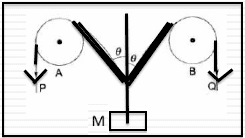# Kinematics, resolving vectors

## Homework Statement

In the arrangement as shown in the figure below, the ends P and Q of an inextensible string move downwards with uniform speed u. Pulleys A and B are fixed. Mass M moves upward with a speed:

a. 2ucosθ
b. u/cosθ
c. 2u/cosθ
d. ucosθ## Homework Equations

None, I am not sure whether it falls under general physics or homework section. [/B]

## The Attempt at a Solution

The strings move with velocity u in their respective directions , thus block will move with velocity 2ucosθ. Since each of the two strings has velocity in vertical direction as ucosθ .

But the answer says it is u/cosθ . Which seems right as when you take components of this total velocity of block M in directions of strings, it gives components as u, but those 2 components don't add up to 2ucosθ. What is the mistake here ? Why are they not adding up to ucosθ?

Thank you for all the support and help.

Orodruin
Staff Emeritus
Homework Helper
Gold Member
The strings are not moving along their transversal direction. They are getting shorter at a given rate. Based on trigonometry, you can find how much shorter the strings become when the mass moves up a certain distance.

•Prannoy Mehta
TSny
Homework Helper
Gold Member
The point where the strings meet moves upward at the unknown speed v. What must be the component of this velocity in a direction parallel to one of the sloping strings?

A small correction to the problem the final answer is u/cosθ. It's very weird.

I din't get how it not being transversal effects this relation, clearly. If I suppose manage to stop time, after recording the instantaneous speeds of both the strings(Since they are at the same angle and move with the same velocity, I will assume it to be u). They both will move with u in their respective directions, and their vertical components will add up, and result in 2ucosθ. And also, if I take the angle approaching 90. The final answer yields in the velocity of it going upward with a velocity tending to infinity, which is not true. And at 90, it should be zero in reality, which the equation I got perfectly relates whereas the answer yields it as infinity, which by any standards is wrong.

Orodruin
Staff Emeritus